# Newton potential

(diff) ← Older revision | Latest revision (diff) | Newer revision → (diff)

A potential with Newton kernel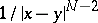, whereis the distance between two pointsandof the Euclidean space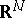,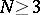, that is, an integral of the form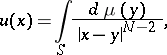(1)

where integration is with respect to a certain Radon measureonwith compact support. When the measureis non-negative, the Newton potential (1) is a superharmonic function in the whole space(see Subharmonic function).

Outside the supportofthe Newton potential (1) has derivatives of all orders in the coordinates ofand is a regular solution of the Laplace equation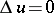, that is,is a harmonic function on the open set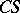and is regular at infinity with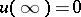. Whenis absolutely continuous, thenhas the form(2)

where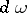is the volume element inandis a certain bounded domain. If here the densityis Hölder continuous in the closed domainand if the boundary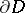consists of finitely many closed Lyapunov hypersurfaces (cf. Lyapunov surfaces and curves), thenhas continuous second-order derivatives insideand satisfies the Poisson equation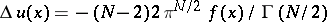.

In Newton's work the concept of a "potential" does not yet occur. The existence of a force function for Newtonian gravitational forces was first proved by J.L. Lagrange in 1773. The terms "potential function" and "potential" applied to integrals of the form (2) for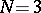were first used by G. Green in 1828 and C.F. Gauss in 1840. The term "Newton potential" is sometimes used in the narrow sense, applied only to volume potentials of the form (2), and sometimes only to the physically real case of a potential (2) of gravitational forces for, created by masses distributed inwith density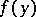.

If an integral of type (2) or (1) is over a hypersurface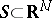, that is, if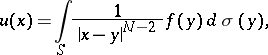(3)

then one speaks of a simple-layer Newton potential; it is a regular harmonic function everywhere outside. Ifis a closed Lyapunov hypersurface and the densityis Hölder continuous on, then the simple-layer Newton potential is continuous everywhere on, and its derivatives are continuous outside. Moreover, its normal derivative in the direction of the outward normaltoat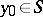has different limits on approachingfrom the inside and the outside. These are expressed by the formulaswhere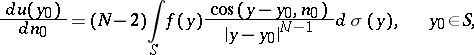is the so-called direct value of the normal derivative of the simple-layer Newton potential, and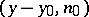is the angle between the vector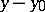and the normal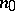; the normal derivative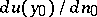is continuous on.

A double-layer Newton potential has the form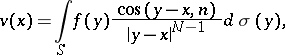(4)

whereis the outward normal toat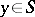. It is also a harmonic function outside, but upon approachingit has a discontinuity. Under the same assumptions onandit has limits from the inside and the outside of. These are expressed by the formulaswhere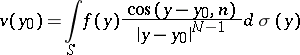is the so-called direct value of the double-layer Newton potential at. Under somewhat more stringent conditions onandthe normal derivative of the double-layer Newton potential is, however, continuous on passing through.

How to Cite This Entry:
Newton potential. Encyclopedia of Mathematics. URL: http://encyclopediaofmath.org/index.php?title=Newton_potential&oldid=11686
This article was adapted from an original article by E.D. Solomentsev (originator), which appeared in Encyclopedia of Mathematics - ISBN 1402006098. See original article